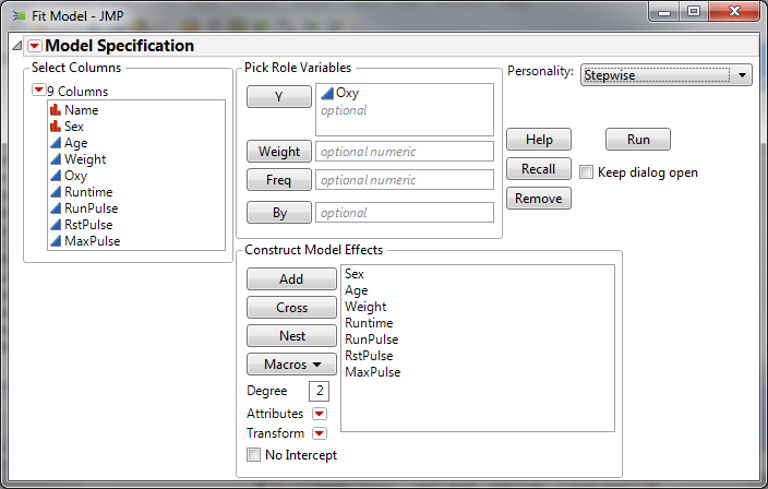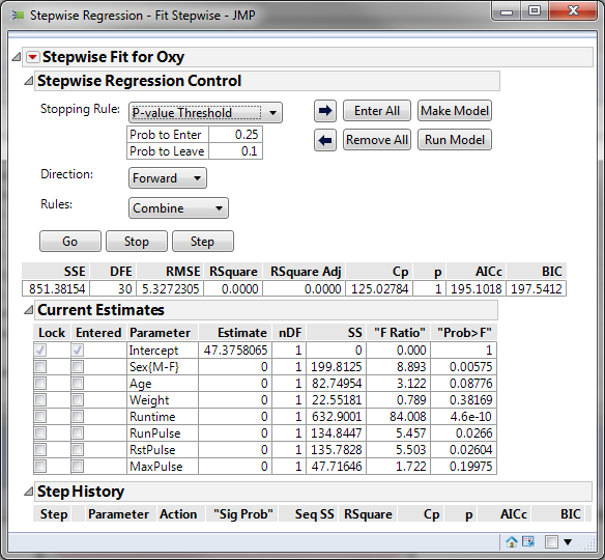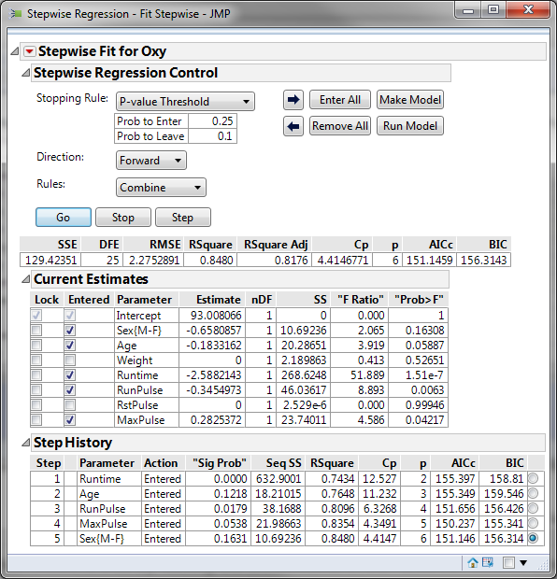# Stepwise Regression with JMP

### What is Stepwise Regression?

Stepwise regression is a statistical method to automatically select regression models with the best sets of predictive variables from a large set of potential variables. There are different statistical methods used in stepwise regression to evaluate the potential variables in the model:

• F-test
• T-test
• R-square
• AIC

### Three Approaches to Stepwise Regression

• Forward Selection
Bring in potential predictors one by one and keep them if they have a significant impact on improving the model.
• Backward Selection
Try out potential predictors individually and eliminate them if they are insignificant to improve the fit.
• Mixed Selection
Is a combination of both forward selection and backward selection. Add and remove variables based on pre-defined significance threshold levels.

### How to Use JMP to Run a Stepwise Regression

Case study: We want to build a regression model to predict the oxygen uptake of a person who runs 1.5 miles. The potential predictors are:

• Age
• Weight
• Runtime
• Runpulse
• RstPulse
• MaxPulse

Data File: “Stepwise Regression.jmp”

#### Run Stepwise Regression in JMP:

1. Click Analyze -> Fit Model
2. Model Specification window appears.
3. Select “Oxy” as the Y and add the potential factors to the model effects boxSelect “Stepwise” in the “Personality” dropdown box
4. Click “Run Mode
5. The “Stepwise Fit” page shows up6. Select the P-value Threshold for Stopping Rule
7. Enter the “Prob to Enter” and “Prob to leave” thresholds into the corresponding text boxes.
8. Select the stepwise regression direction
• Forward
• Backward
• Mixed
1. Click the “Go” button to let JMP automatically find the set of predictors satisfying the pre-defined significance probability thresholds.Model summary: Two of the seven potential factors are not statistically significant since their p-value is higher than the alpha to enter. Step History: Step-by-step records on how to develop the final model. Each column indicates the model built in each step.